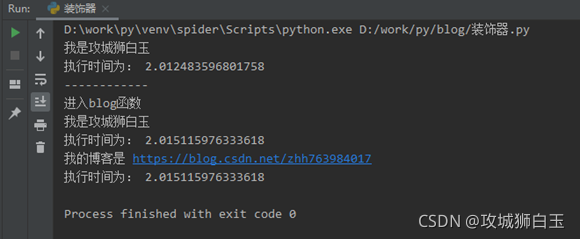# python裝飾器原理源碼示例分析

win10

python3.7

## 二、為什麼要用裝飾器

```def baiyu():
print("我是攻城獅白玉")
def blog(name):
print('進入blog函數')
name()
print('我的博客是 https://blog.csdn.net/zhh763984017')
if __name__ == '__main__':
func = baiyu  # 這裡是把baiyu這個函數名賦值給變量func
func()  # 執行func函數
print('------------')
blog(baiyu)  # 把baiyu這個函數作為參數傳遞給blog函數```接下來，我想知道這`baiyu``blog`兩個函數分別的執行時間是多少，我就把代碼修改如下：

```import time
def baiyu():
t1 = time.time()
print("我是攻城獅白玉")
time.sleep(2)
print("執行時間為：", time.time() - t1)
def blog(name):
t1 = time.time()
print('進入blog函數')
name()
print('我的博客是 https://blog.csdn.net/zhh763984017')
print("執行時間為：", time.time() - t1)
if __name__ == '__main__':
func = baiyu  # 這裡是把baiyu這個函數名賦值給變量func
func()  # 執行func函數
print('------------')
blog(baiyu)  # 把baiyu這個函數作為參數傳遞給blog函數```上述的改寫已經實現瞭我需要的功能，但是，當我有另外一個新的函數【python_blog_list】，具體如下：

```def python_blog_list():
print('''【Python】爬蟲實戰，零基礎初試爬蟲下載圖片（附源碼和分析過程）
https://blog.csdn.net/zhh763984017/article/details/119063252 ''')
print('''【Python】除瞭多線程和多進程，你還要會協程
https://blog.csdn.net/zhh763984017/article/details/118958668 ''')
print('''【Python】爬蟲提速小技巧，多線程與多進程（附源碼示例）
https://blog.csdn.net/zhh763984017/article/details/118773313 ''')
print('''【Python】爬蟲解析利器Xpath，由淺入深快速掌握（附源碼例子）
https://blog.csdn.net/zhh763984017/article/details/118634945 ''')```

```def python_blog_list():
t1 = time.time()
print('''【Python】爬蟲實戰，零基礎初試爬蟲下載圖片（附源碼和分析過程）
https://blog.csdn.net/zhh763984017/article/details/119063252 ''')
print('''【Python】除瞭多線程和多進程，你還要會協程
https://blog.csdn.net/zhh763984017/article/details/118958668 ''')
print('''【Python】爬蟲提速小技巧，多線程與多進程（附源碼示例）
https://blog.csdn.net/zhh763984017/article/details/118773313 ''')
print('''【Python】爬蟲解析利器Xpath，由淺入深快速掌握（附源碼例子）
https://blog.csdn.net/zhh763984017/article/details/118634945 ''')
print("執行時間為：", time.time() - t1)```## 三、簡單的裝飾器

```import time
def baiyu():
print("我是攻城獅白玉")
time.sleep(2)
def count_time(func):
def wrapper():
t1 = time.time()
func()
print("執行時間為：", time.time() - t1)
return wrapper
if __name__ == '__main__':
baiyu = count_time(baiyu)  # 因為裝飾器 count_time(baiyu) 返回的時函數對象 wrapper，這條語句相當於  baiyu = wrapper
baiyu()  # 執行baiyu()就相當於執行wrapper()```

## 四、裝飾器的語法糖

baiyu = count_time(baiyu)

```import time
def count_time(func):
def wrapper():
t1 = time.time()
func()
print("執行時間為：", time.time() - t1)
return wrapper
@count_time
def baiyu():
print("我是攻城獅白玉")
time.sleep(2)
if __name__ == '__main__':
# baiyu = count_time(baiyu)  # 因為裝飾器 count_time(baiyu) 返回的時函數對象 wrapper，這條語句相當於  baiyu = wrapper
# baiyu()  # 執行baiyu()就相當於執行wrapper()
baiyu()  # 用語法糖之後，就可以直接調用該函數瞭```

## 五、裝飾器傳參

```def blog(name):
print('進入blog函數')
name()
print('我的博客是 https://blog.csdn.net/zhh763984017')```

```def count_time(func):
def wrapper(*args,**kwargs):
t1 = time.time()
func(*args,**kwargs)
print("執行時間為：", time.time() - t1)
return wrapper```

```import time
def count_time(func):
def wrapper(*args, **kwargs):
t1 = time.time()
func(*args, **kwargs)
print("執行時間為：", time.time() - t1)
return wrapper
@count_time
def blog(name):
print('進入blog函數')
name()
print('我的博客是 https://blog.csdn.net/zhh763984017')
if __name__ == '__main__':
# baiyu = count_time(baiyu)  # 因為裝飾器 count_time(baiyu) 返回的時函數對象 wrapper，這條語句相當於  baiyu = wrapper
# baiyu()  # 執行baiyu()就相當於執行wrapper()

# baiyu()  # 用語法糖之後，就可以直接調用該函數瞭
blog(baiyu)```

## 六、帶參數的裝飾器

```import time
def count_time_args(msg=None):
def count_time(func):
def wrapper(*args, **kwargs):
t1 = time.time()
func(*args, **kwargs)
print(f"[{msg}]執行時間為：", time.time() - t1)
return wrapper
return count_time
@count_time_args(msg="baiyu")
def fun_one():
time.sleep(1)
@count_time_args(msg="zhh")
def fun_two():
time.sleep(1)
@count_time_args(msg="mylove")
def fun_three():
time.sleep(1)
if __name__ == '__main__':
fun_one()
fun_two()
fun_three()```## 七、類裝飾器

• 通過__init__（）方法初始化類
• 通過__call__（）方法調用真正的裝飾方法
```import time
class BaiyuDecorator:
def __init__(self, func):
self.func = func
print("執行類的__init__方法")
def __call__(self, *args, **kwargs):
print('進入__call__函數')
t1 = time.time()
self.func(*args, **kwargs)
print("執行時間為：", time.time() - t1)
@BaiyuDecorator
def baiyu():
print("我是攻城獅白玉")
time.sleep(2)
def python_blog_list():
time.sleep(5)
print('''【Python】爬蟲實戰，零基礎初試爬蟲下載圖片（附源碼和分析過程）
https://blog.csdn.net/zhh763984017/article/details/119063252 ''')
print('''【Python】除瞭多線程和多進程，你還要會協程
https://blog.csdn.net/zhh763984017/article/details/118958668 ''')
print('''【Python】爬蟲提速小技巧，多線程與多進程（附源碼示例）
https://blog.csdn.net/zhh763984017/article/details/118773313 ''')
print('''【Python】爬蟲解析利器Xpath，由淺入深快速掌握（附源碼例子）
https://blog.csdn.net/zhh763984017/article/details/118634945 ''')
@BaiyuDecorator
def blog(name):
print('進入blog函數')
name()
print('我的博客是 https://blog.csdn.net/zhh763984017')
if __name__ == '__main__':
baiyu()
print('--------------')
blog(python_blog_list)```## 八、帶參數的類裝飾器

```class BaiyuDecorator:
def __init__(self, arg1, arg2):  # init()方法裡面的參數都是裝飾器的參數
print('執行類Decorator的__init__()方法')
self.arg1 = arg1
self.arg2 = arg2
def __call__(self, func):  # 因為裝飾器帶瞭參數，所以接收傳入函數變量的位置是這裡
print('執行類Decorator的__call__()方法')
def baiyu_warp(*args):  # 這裡裝飾器的函數名字可以隨便命名，隻要跟return的函數名相同即可
print('執行wrap()')
print('裝飾器參數：', self.arg1, self.arg2)
print('執行' + func.__name__ + '()')
func(*args)
print(func.__name__ + '()執行完畢')
return baiyu_warp
@BaiyuDecorator('Hello', 'Baiyu')
def example(a1, a2, a3):
print('傳入example()的參數：', a1, a2, a3)
if __name__ == '__main__':
print('準備調用example()')
example('Baiyu', 'Happy', 'Coder')
print('測試代碼執行完畢')```

## 九、裝飾器的順序

```def BaiyuDecorator_1(func):
def wrapper(*args, **kwargs):
func(*args, **kwargs)
print('我是裝飾器1')
return wrapper
def BaiyuDecorator_2(func):
def wrapper(*args, **kwargs):
func(*args, **kwargs)
print('我是裝飾器2')
return wrapper
def BaiyuDecorator_3(func):
def wrapper(*args, **kwargs):
func(*args, **kwargs)
print('我是裝飾器3')
return wrapper
@BaiyuDecorator_1
@BaiyuDecorator_2
@BaiyuDecorator_3
def baiyu():
print("我是攻城獅白玉")
if __name__ == '__main__':
baiyu()``````@BaiyuDecorator_1
@BaiyuDecorator_2
@BaiyuDecorator_3
def baiyu():
print("我是攻城獅白玉")```

baiyu = BaiyuDecorator_1 (BaiyuDecorator_2 (BaiyuDecorator_3(baiyu)))